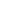# Math Plasticine

Math Plasticine is a fun game in which you can test your knowledge of a science such as math. In front of the screen is a playing field with a mathematical equation. A question mark will appear at the end. There will be numbers in the equation on special cells.

Examine the equation carefully and solve it mentally. Then, using your mouse, select one of the numbers printed on the tile. You will respond in this manner. If you answer correctly, you will be awarded points and advance to the next level of the Math Plasticine game. If the answer is incorrect, you will fail. Have a great time!

You can join many similar games with this game like Water Lab. Share the most interesting game moments.

Instruction to play